# GSMLine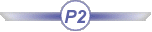## Definition:

A GSMLine is a line :

• generated by the Generative Shape Design product.
• available in the BasicWireFrame Package.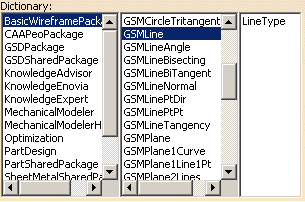## Attributes:

###### LineType

A line is defined by its type. The attribute to be used is LineType. The syntax to be used is: LineType = i, i corresponding to the type of line that you want to create.
Please find below an equivalence table listing the existing types of lines that you can create and the digit to indicate.
 Line Type in GSD Line Type in the Package Corresponding digit Point to Point GSMLinePtPt 0 Point-Direction GSMLinePtDir 1 Angle to Curve GSMLineAngle 2 Tangent to Curve GSMLineTangency 3 Normal to surface GSMLineNormal 4 Intersection betw. 2 planes GSMLineBiTangent 5

As mentioned above, you may create 7 different line sub-types. Please find below a description of each sub-type, as well as its attributes and the syntax to use.

### Point to Point Line (GSMLinePtpt)

The sub-type to be used in this case is GSMLinePtpt which defines the line extremities. The following attributes are available for this sub-type:

 FirstPoint (feature) SecondPoint  (feature) Support (feature) Length1 (length, optional for both combinations) Length2 (length, optional for both combinations)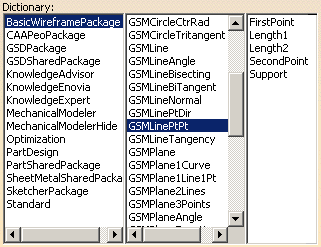These attributes can be combined as follows:

1st combination 2nd combination
• the FirstPoint which is defined by the syntax below:
`FirstPoint = object: ..\..\theFirstPoint``;`
• the SecondPoint which is defined by the syntax below:
`SecondPoint = object: ..\..\theSecondPoint``;`
• Length1 which is defined by the syntax below:
Length1=200mm;
• Length2 which is defined by the syntax below:
Length2=150mm;
• the FirstPoint which is defined by the syntax below:
`FirstPoint = object: ..\..\theFirstPoint``;`
• the SecondPoint which is defined by the syntax below:
`SecondPoint = object: ..\..\theSecondPoint``;`
• the Support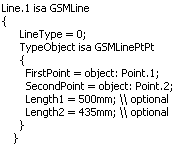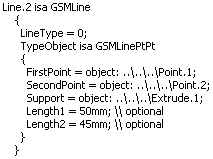### Point-Direction (GSMLinePtDir)

The sub-type to be used in this case is GSMLinePtDir which defines the line direction. The following attributes are available for this sub-type:

 Length1 Length2 Direction Orientation RefPoint Support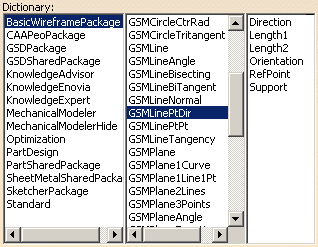These attributes can be combined as follows:

Combination
• Length1 which is defined by the syntax below:
`Length1`` = 100mm;`
• Length2 which is defined by the syntax below:
`Length2`` = 10mm;`
• Direction which is defined by the syntax below:
`Direction`` = object: ..\..\Plane.2``;`
• RefPoint which is defined by the syntax below:
`RefPoint`` = object: ..\..\Point.2``;`
• Support which is defined by the syntax below:
```SecondPoint = object: ..\..\'xy plane'````;`

### Tangent to Curve (GSMLineTangency)

The sub-type to be used in this case is GSMLineTangency. The following attributes are available for this sub-type:

 Curve: Reference curve used to define the tangency. Length1 Length2 Orientation RefPoint: Reference point used to define the tangency. Support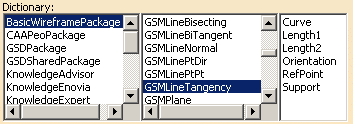These attributes can be combined as follows:

Combination
• Curve which is defined by the syntax below:
`Curve`` = object: ..\..\Spline.2;`
• Length1 which is defined by the syntax below:
`Length1`` = 100mm;`
• Length2 which is defined by the syntax below:
`Length2`` = 10mm;`
• RefPoint which is defined by the syntax below:
`RefPoint`` = object: ..\..\Point.2;`
• Support which is defined by the syntax below:
```SecondPoint = object: ..\..\'xy plane'````;`

### Normal to surface (GSMLineNormal)

The sub-type to be used in this case is GSMLineNormal. The following attributes are available for this sub-type:

 Orientation RefPoint RefSkin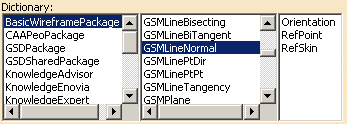These attributes can be combined as follows:

Combination
• RefPoint which is defined by the syntax below:
`RefPoint`` = object: ..\..\Point.2;`
• Support which is defined by the syntax below:
`RefSkin`` = object: ..\..\Extrude.1``;`